Next: 1.4 Using the shift Up: 1 Multiple Precision Arithmetic Previous: 1.2 Multiplication Algorithm

## 1.3 Long Division Algorithm

This is by far the most complicated procedure. The first point to note is that the school method for long division involves a guess''. We need to ensure that this guess is replaced a multiple-choice procedure (also characterised by a case'' statement). Moreover, the number of choices should be very small and not of the same size as the base M.

As for the case of multiplication we can understand some aspects of the relevant book-keeping by performing the short'' division

(v1 ... vq) = u×(w1 ... wq) + t

where t < u. We first compute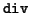(u, 0, v1) = (w1, t1). From then on we have the inductive approach(u, ti, vi + 1) = (wi + 1, ti + 1). Clearly, the first step is a special case of the second by putting v0 = 0. At the end t = tq. Moreover, there are exactly q steps.

There are two procedures for long division, each with its own level of complexity. We note that in the division

(v0 ... vq) = (u1 ... uqw + (t1 ... tq)

where v0 < u1 and (t1 ... tq) < (u1 ... uq), a good guess'' for w is provided by x which is obtained by(u1, v0, v1) = (x, y) (so that y < u1). The following lemma tells us just how good the guess is

Lemma 1   If 2 . u1 + 1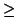M then we have x - 2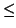wx.

Proof. Using (u2 ... uq)Mq - 1 - 1 and (v2 ... vq)Mq - 1 we obtain the following inequalities
 u1 . Mq - 1(u1 ... uq)u1Mq - 1 + Mq - 1 - 1 (v0 . M + v1) . Mq - 1(v0 ... vq)(v0 . M + v1) . Mq - 1 + Mq - 1 - 1

which we can combine with the Euclidean inequalities
 u1 . xv0 . M + v1u1 . (x + 1) - 1 (u1 ... uq)×w(v0 ... vq)(u1 ... uq)×(w + 1) - 1

We then obtain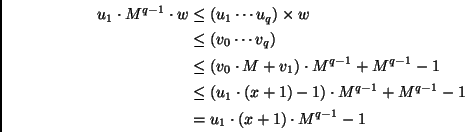As a consequence w < (x + 1) or equivalently (since these are integers) wx. The reverse comparison is provided by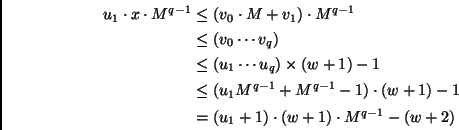It follows that u1 . x < (u1 + 1) . (w + 1) or (since we have integers on both sides) u1 . xu1 . (w + 1) + w. Now the condition v0 < u1 implies that x(M - 1). So if wM - 1, then we certainly have the inequality xw + 2 as required. Thus we may assume that w < M - 1. From the assumption 2 . u1M - 1 we obtain w < u1 . 2. Combining this with the above we see that

u1 . xu1 . (w + 1) + w < u1 . (w + 3)

or equivalently xw + 2 as required.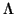Using this lemma, we see that we can give an algorithm for the long division

(v1 ... vp) = (u1 ... uq)×(w0 ... wr) + (t1 ... ts)

that involves making one out of three choices. In order to apply the lemma we must first normalise the divisor (u1 ... uq). So as a first step we compute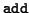(u1, 1, 0) = (t,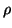). If= 1, then we take d = 1, other wise we compute(t, 1, 0) = (d, r). Thus in every case we have d is the integer part of M/(u1 + 1). We now take
 (x1 ... xq) = d×(u1 ... uq) (y0 ... yp) = d×(v1 ... vp)

Here we take y0 = 0 if necessary. We check easily that this step also ensures that y0 < x1. Next we initialise (t0, 0 ... t0, q - 1) = (y0 ... yq - 1). We can then perform the long division by induction as follows. Let(x1, ti, 0, ti, 1) = (gi, r); then gi is our guess. We compute (and note that the z sequence cannot be longer than q due to the choice of gi)

(ti, 0 ... ti, q - 1yq + i) - gi×(x1 ... xq) = (zi, 0 ... zi, q - 1) -. Mq

If= 0 then we take (ti + 1, 0 ... ti + 1, q - 1) = (zi, 0 ... zi, q - 1) and wi = gi. Otherwise (we have= 1 and) we compute

(zi, 0 ... zi, q - 1) + (x1 ... xq) = (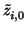...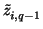) +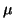. Mq

Now, if= 1 then we take (ti + 1, 0 ... ti + 1, q - 1) = (...) and(gi, 1, 0) = (wi, 0). Finally, if we have= 0, then we put

(...) + (x1 ... xq) = (ti + 1, 0 ... ti + 1, q - 1) +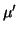. Mq

We note that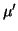= 1 is ensured by the lemma above. We put(gi, 2, 0) = (wi, 0) in this case. After r = p - q steps we obtain the identity

(y0 ... yp) = (x1 ... xq)×(w0 ... wr) + (tr + 1, 0 ... tr + 1, q - 1)

From the choices for x's and y's we see that d exactly divides the remainder, so we perform short division to obtain

(tr + 1, 0 ... tr + 1, q - 1) = d×(t1 ... tq)

This completes the long division which takes about 3rq steps upto terms linear in p and q (such as additions and subtractions).

Another way to simplify the guessing process is to take (u0u1 ... uq) of the form (10u2 ... uq). In this case, the division of (v0 ... vq) always yields one of v0 or v0 - 1 as the quotient. The main question is then how to perform the necessary normalisation (to bring the u into this form). Starting with (u1 ... uq) we first compute(u1, 1, 0) = (t,) and if= 1 we put d = 1. Otherwise, we compute(t, 1, 1) = (d, r). Thus, in every case d is the integer part of (M + 1)/(u1 + 1). As before, we multiply by d

 (x1, 0 ... x1, q) = d×(u1 ... uq) (y1, 0 ... y1, p) = d×(v1 ... vp)

Where we put x1, 0 = 0 and y1, 0 = 0 if there is no carry over. Now, if xi, 1 = 0 (then xi, 0 = 1 is forced) we stop. Otherwise, we perform the following steps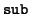(0, x1, 1, 0) = (ci, 1)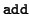(x1, 1), 1, 0) = (ti,) if= 0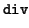(ti, ci, 0) = (di, r) if= 1 di = ci

Thus in every case, we have di is the integer part of M(M - x1, 1)/(xi, i + 1). Now, we multiply by (1.di)
 (xi + 1, 0 ... xi + 1, q.xi + 1, q + 1 ... ) = (1.di)×(xi, 0 ... xi, q.xi, q + 1 ... ) (yi + 1, 0 ... yi + 1, p.yi + 1, q + 1 ... ) = (1.di)×(yi, 0 ... yi, q.yi, q + 1 ... )

where we use the (.) to keep the notation simple and note that there are only finitely many terms after it. We now iterate over i.

Lemma 2   For some i3 we obtain (xi, 0xi, 1xi, 2 ... ) = (10xi, 2 ... ) as required.

Assuming this for the moment we see that have removed alloperations in the iterative part of the long division process. We leave it to the reader to make an algorithm out of this procedure. The proof of the lemma is a somewhat complicated exercise which we skip as well.Next: 1.4 Using the shift Up: 1 Multiple Precision Arithmetic Previous: 1.2 Multiplication Algorithm
Kapil Hari Paranjape 2002-10-20